﻿ 水下浮筒对于深水系泊缆静态特性的影响
 舰船科学技术2018, Vol. 40Issue (4): 68-72PDF

Influence of the submersible buoy on the static characteristics of mooring cable in deep water
ZHAO Jing-rui, WANG Shi-sheng, FENG Jia-guo
CNOOC Research Institute, Beijing 100027, China
Abstract: A flexible mechanics model of the mooring line is established according the lumped mass method. The effects of the net buoyancy, location and the current force of the buoy on the static characteristics of the mooring line are analyzed. The result shows that by using submersible buoy, the mooring load and pretension will be reduced, and the horizontal stiffness is improved, but the location where the maximum cable tension appears maybe changed. The buoyancy and location of the buoy exist optimum values, the influence of the current force on the buoy is very limited.
Key words: submersible mooring buoy     mooring load     static characteristics     cable tension
0 引　言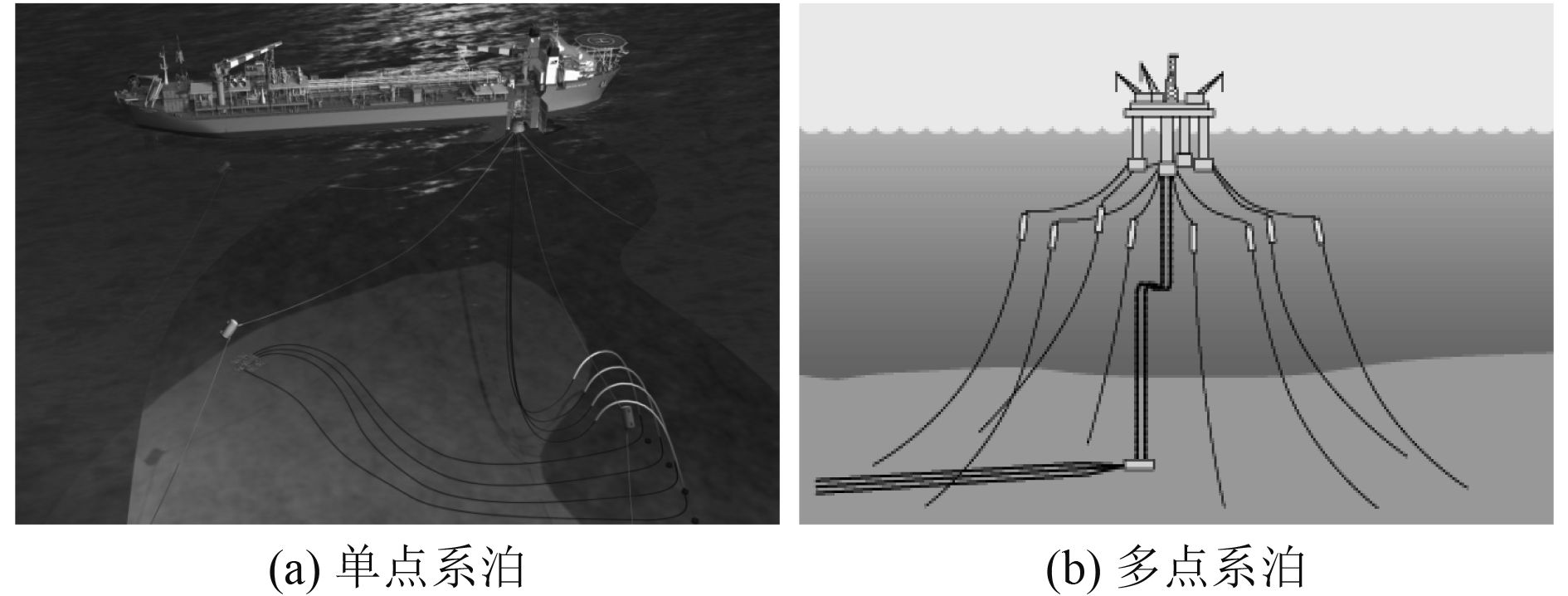图 1 配备水下浮筒的深水浮式平台系泊系统 Fig. 1 Mooring system of floating platform with submergible buoy in deepwater

1 缆绳力学模型的建立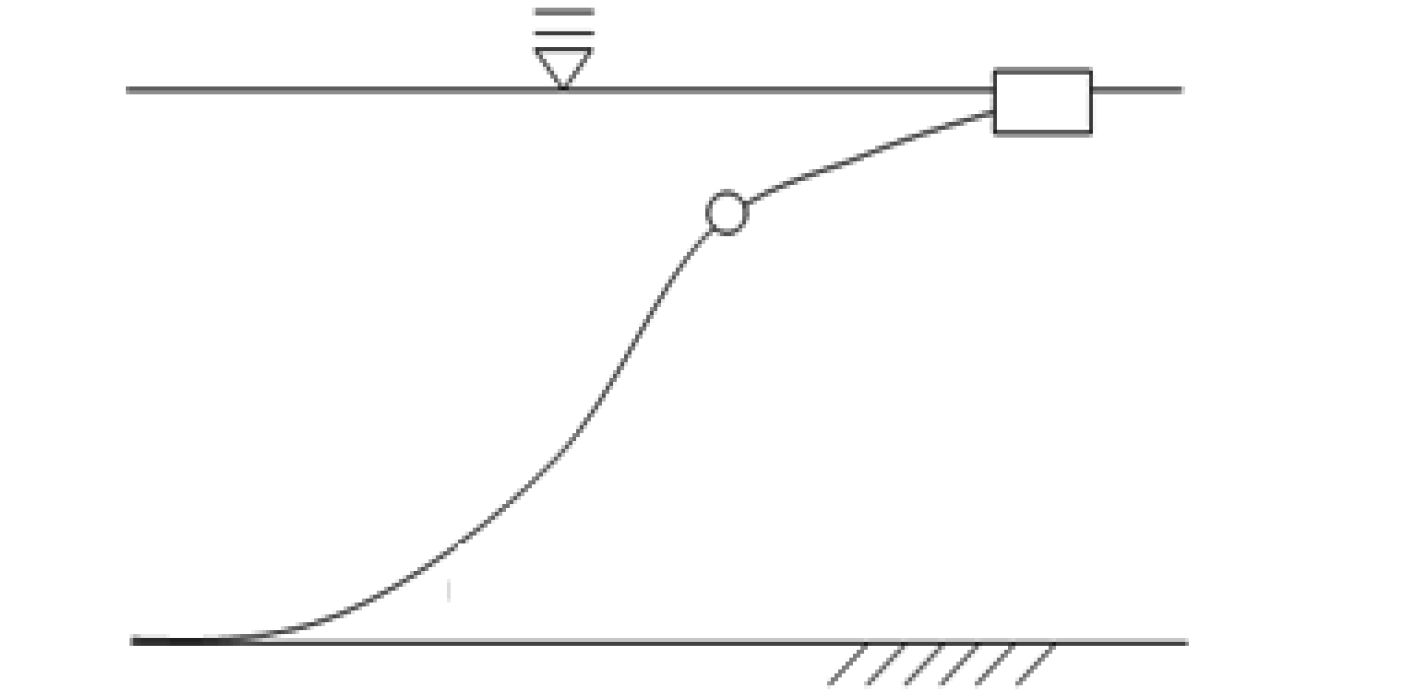图 2 缆绳水中构型 Fig. 2 Configuration of mooring line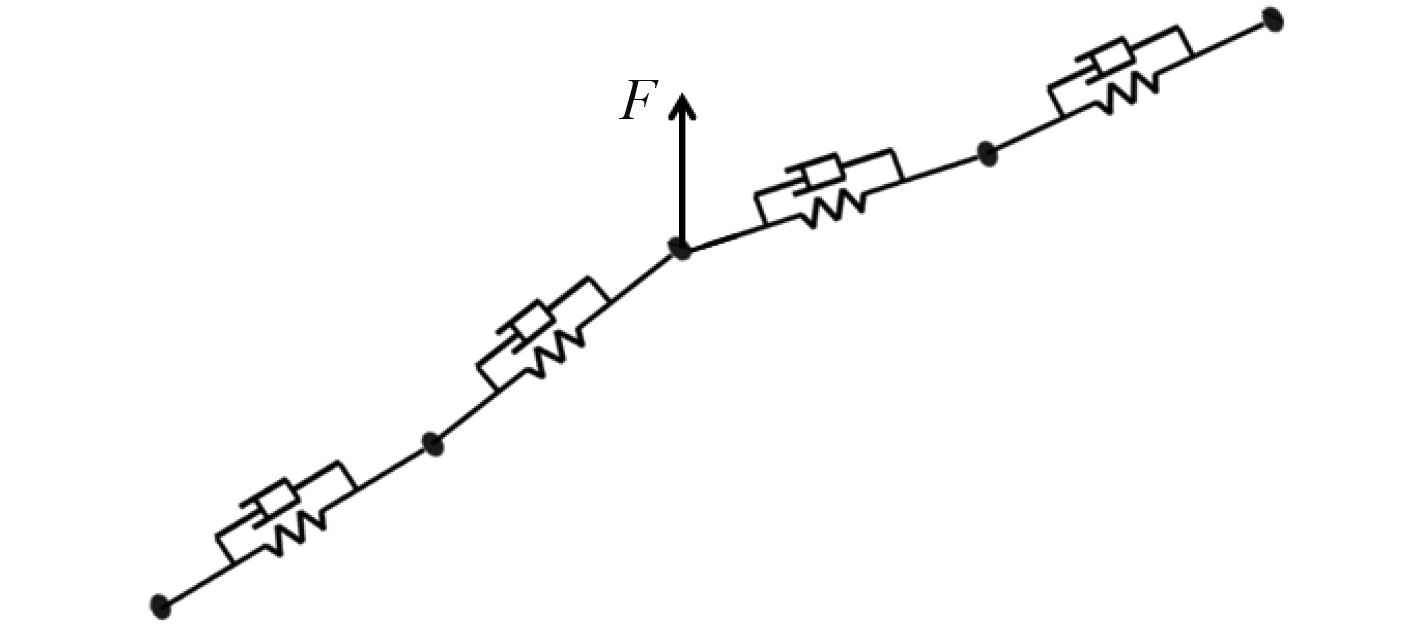图 3 缆绳局部力学模型 Fig. 3 Partial mechanical model of mooring line

 $\left\{ {{F_k}} \right\} = \left\{ {F_{GBT}^k} \right\} + \left\{ {F_T^k} \right\} + \left\{ {F_D^k} \right\} + \left\{ {F_I^k} \right\}\text{。}$ (1)

${p_k}$ 点的等效轴向拉伸回复力与结构内阻尼力 $\left\{ {F_T^k} \right\}$ 可表示为：

 $F_T^k = \left( {F_{TH}^k + F_{TD}^k} \right)\overrightarrow {{t_k}} - \left( {F_{TH}^{k - 1} + F_{TD}^{k - 1}} \right)\overrightarrow {{t_{k - 1}}}\text{。}$ (2)

 $F_{TD}^k = - {\mu _k}\left( {\overrightarrow {{v^k}} - \overrightarrow {{v^{k - 1}}} } \right)\overrightarrow {{t_k}}\text{。}$ (3)

 $\begin{split}\vec F_d^{{l_k}} =& - \frac{1}{2}{\rho _f}A_T^kC_{dt}^k\left| {\overrightarrow {v_r^k} \cdot \overrightarrow {{t_k}} } \right|\left( {\overrightarrow {v_r^k} \cdot \overrightarrow {{t_k}} } \right)\overrightarrow {{t_k}} - \\&\frac{1}{2}{\rho _f}A_N^kC_{dn}^k\left| {\overrightarrow {v_r^k} \cdot \overrightarrow {{h_k}} } \right|\left( {\overrightarrow {v_r^k} \cdot \overrightarrow {{h_k}} } \right)\overrightarrow {{h_k}} \text{。}\end{split}$ (4)

 $\overrightarrow {v_r^k} = \overrightarrow {{v^{sk}}} - \overrightarrow {v_f^{sk}} \text{。}$ (5)

 $\overrightarrow F _I^{{l_k}} = - \frac{1}{4}{\rho _f}\pi {D^2}C_M^k\left( {\overrightarrow {\dot v_f^{sk}} \cdot \overrightarrow {{h_k}} } \right)\overrightarrow {{h_k}} \text{。}$ (6)

 $\overrightarrow F _d^k = \frac{1}{2}\left( {\overrightarrow F _d^{{l_k}} + \overrightarrow F _d^{{l_{k - 1}}} + \overrightarrow F _I^{{l_k}} + \overrightarrow F _I^{{l_{k - 1}}}} \right)\text{。}$ (7)
2 数值算例表 1 单根缆绳结构组成 Tab.1 The composition of mooring line表 2 缆绳分段与节点编号范围 Tab.2 Mooring line component and corresponding envelope of nodes numbering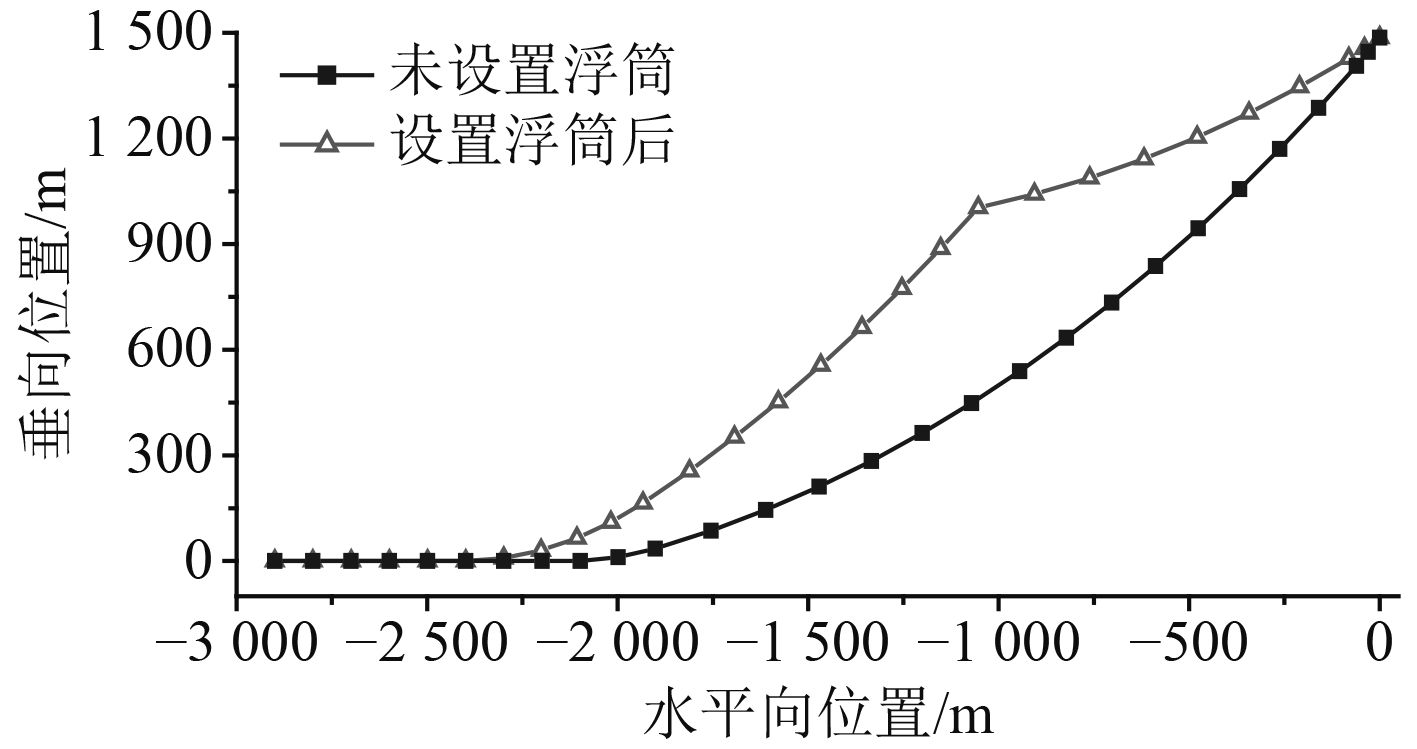图 4 缆绳水中构型对比 Fig. 4 Comparison of mooring line configuration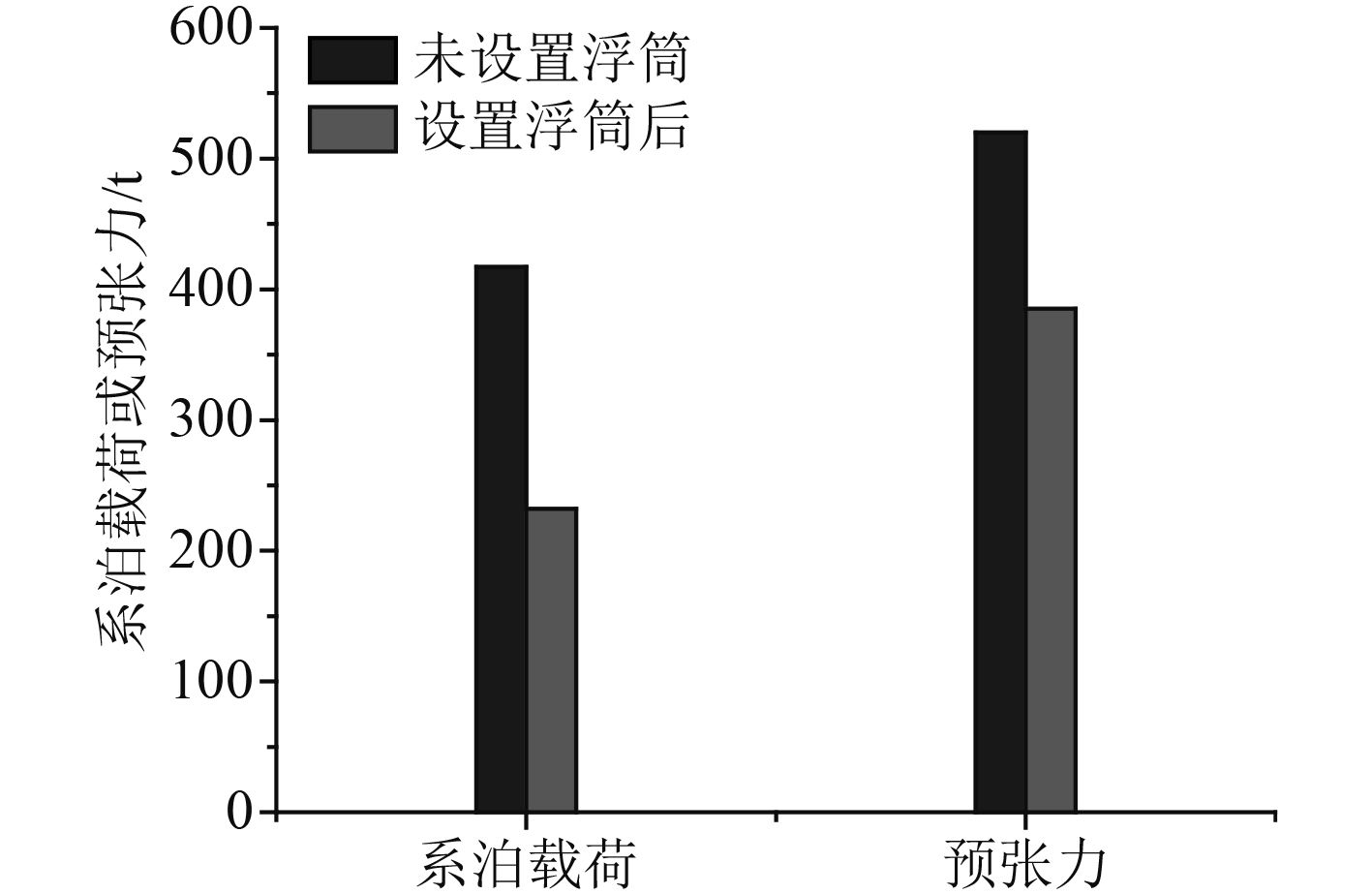图 5 系泊载荷与预张力对比 Fig. 5 Comparison of mooring load and pretension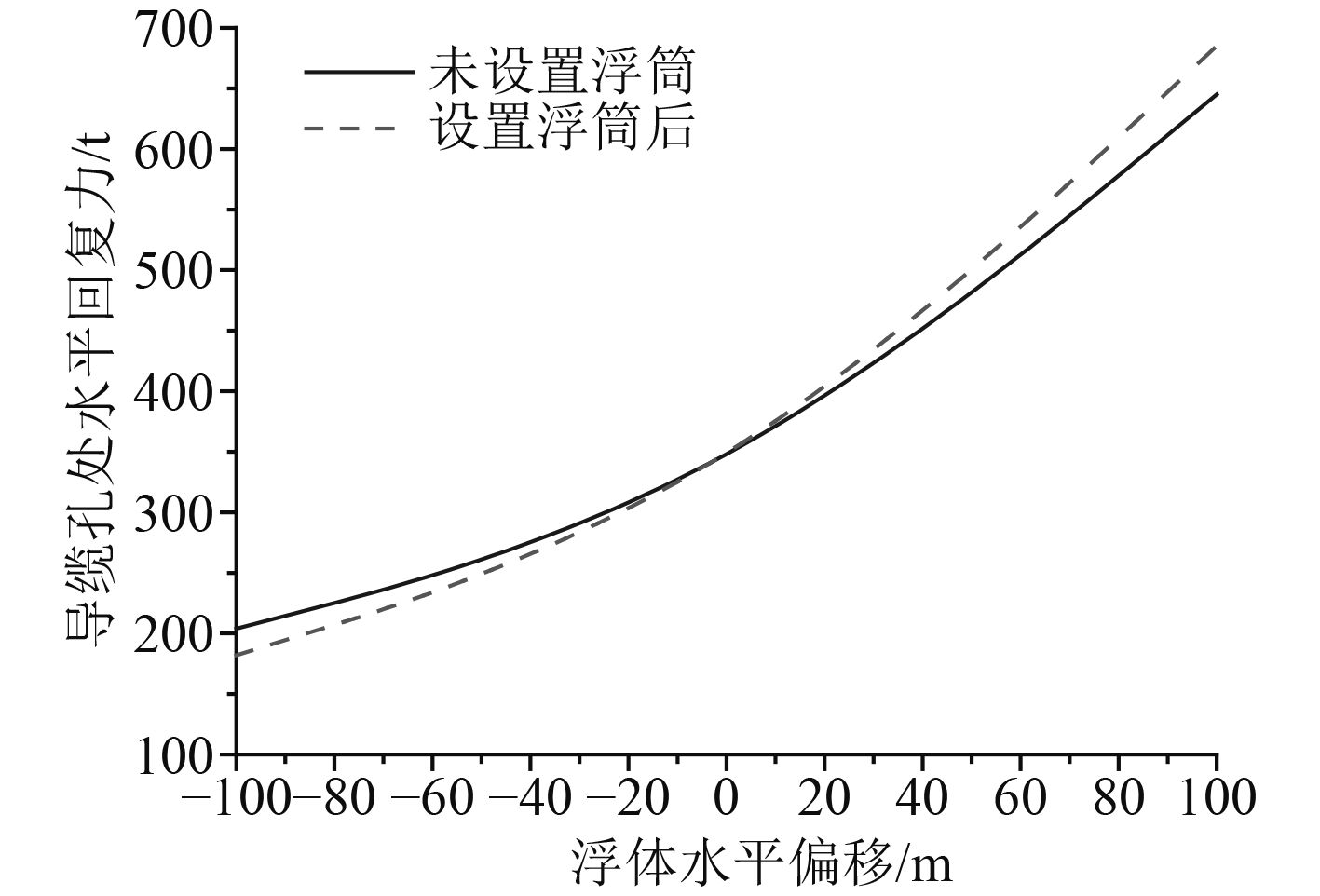图 6 缆绳水平刚度对比 Fig. 6 Comparison of horizontal restoring stiffness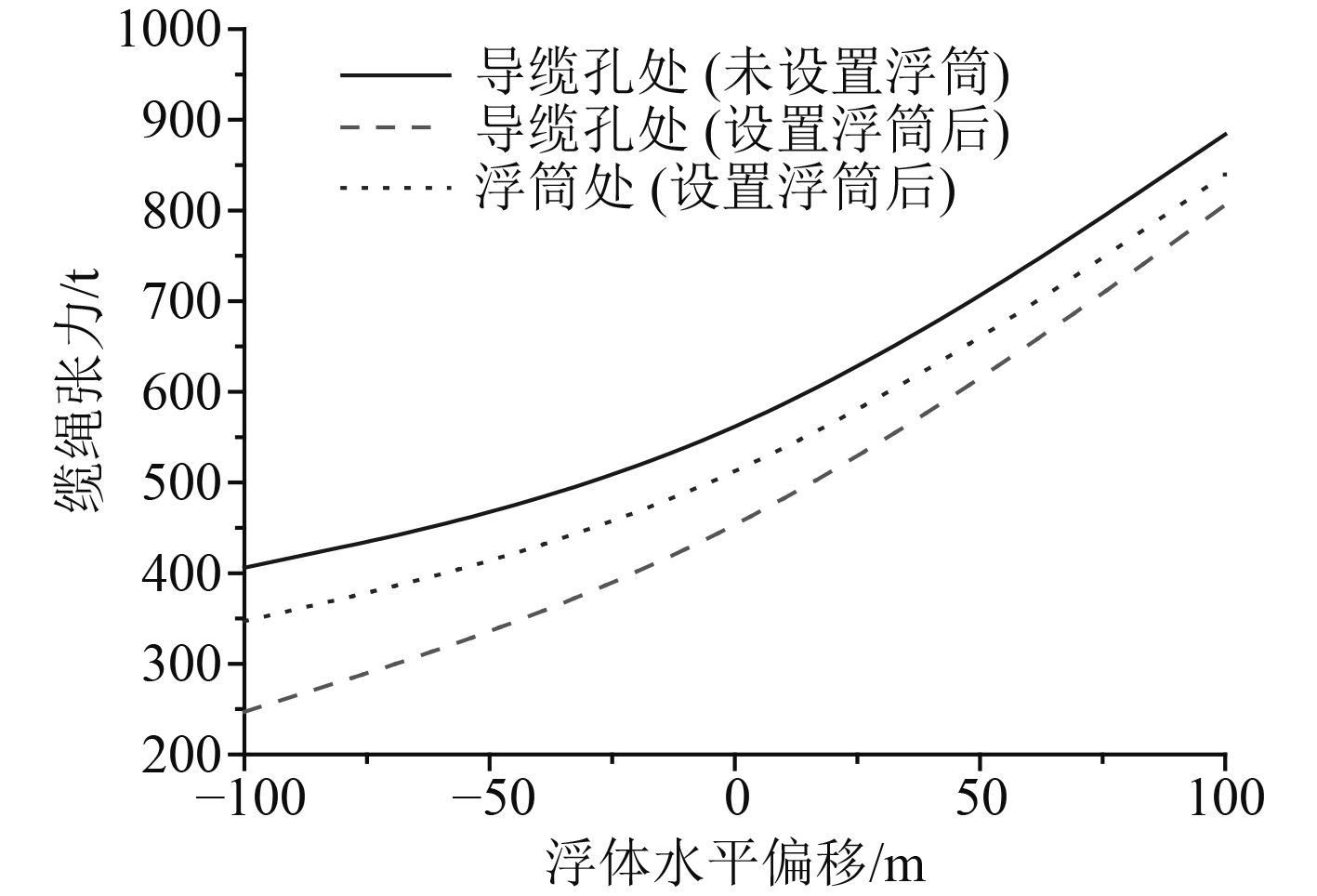图 7 不同位置处缆绳张力对比 Fig. 7 Comparison of tension at different location

2.1 浮筒净浮力的影响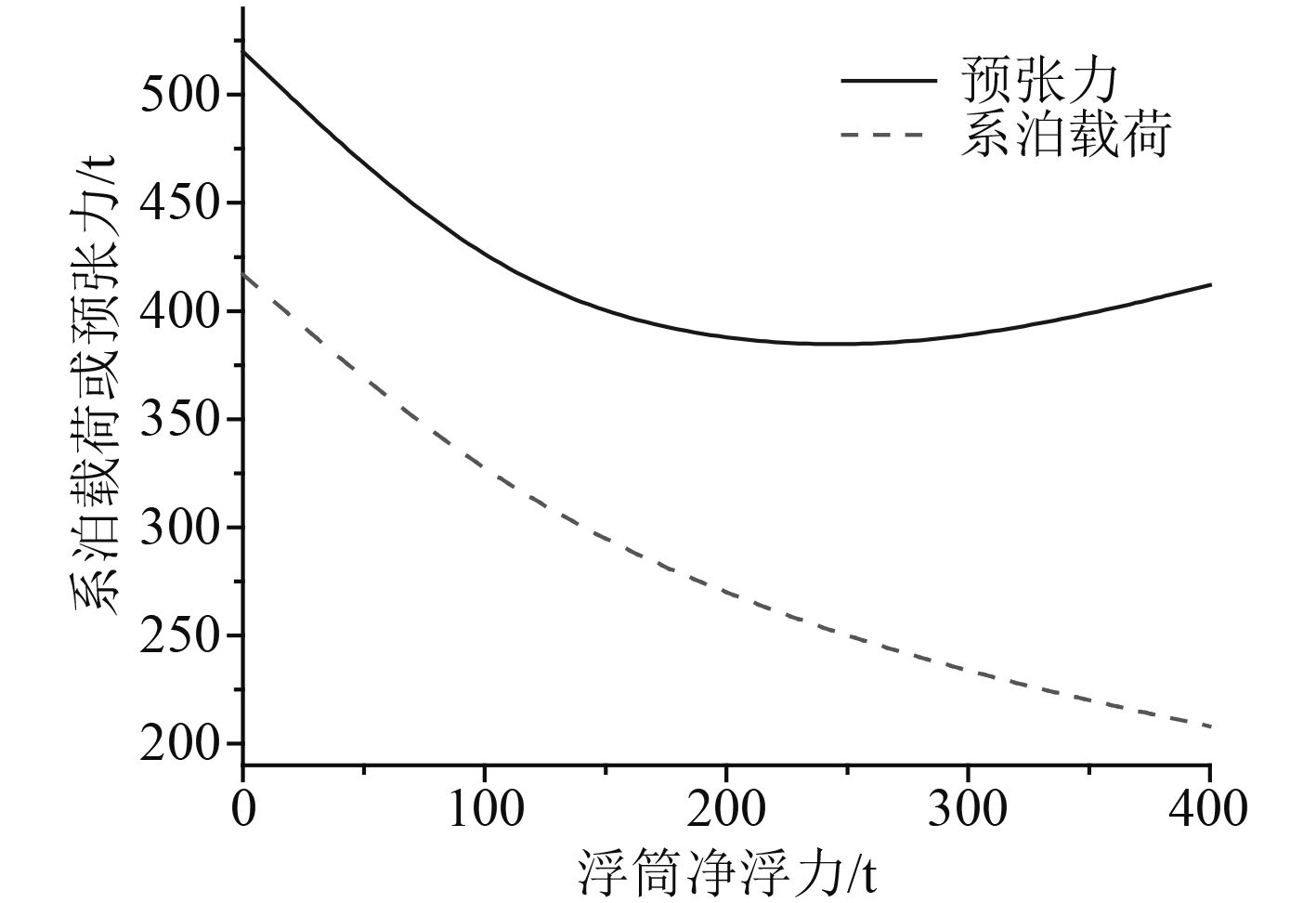图 8 预张力与系泊载荷的变化曲线 Fig. 8 Curves of mooring load and pretension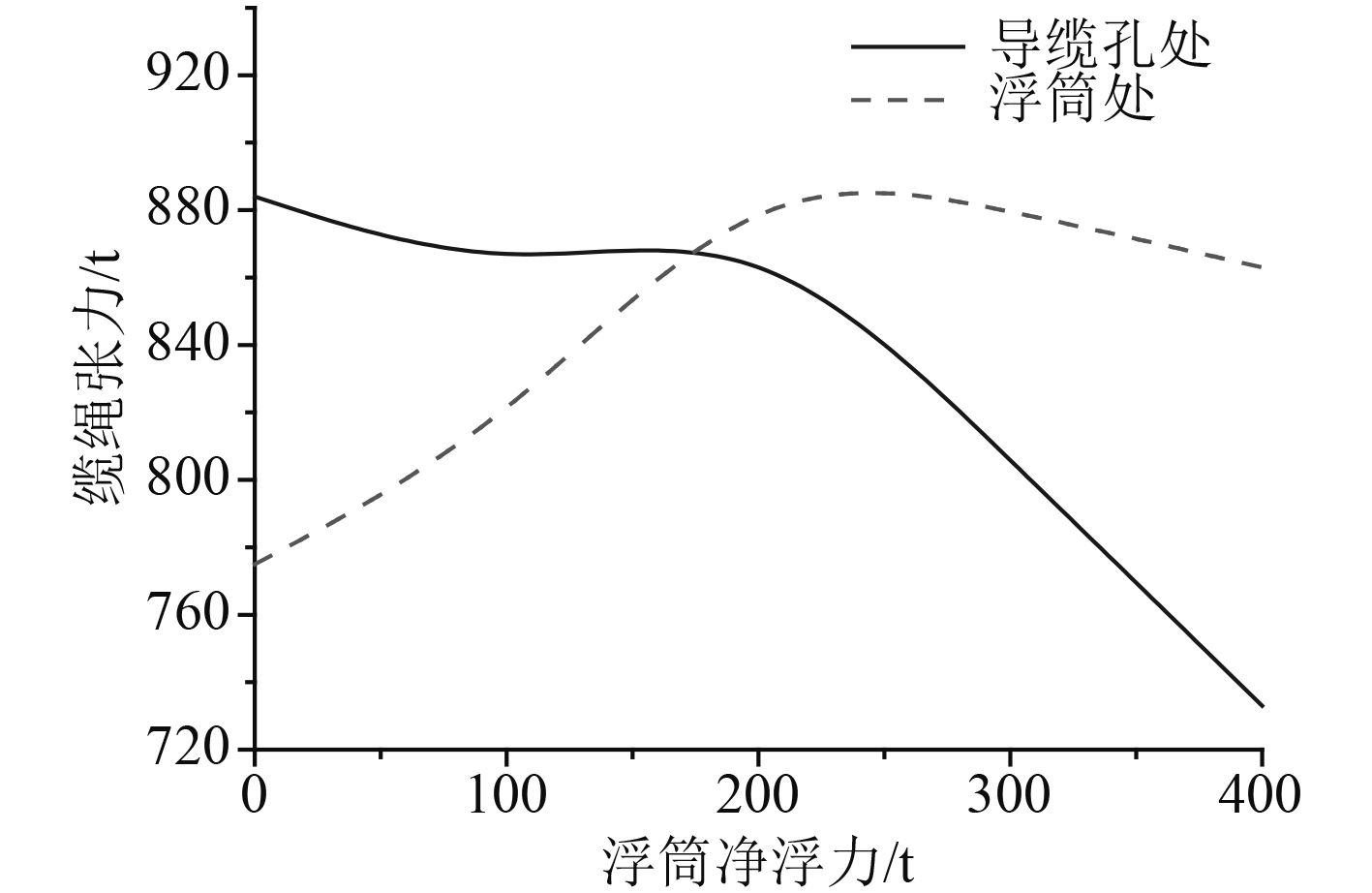图 9 缆绳系泊张力的变化曲线 Fig. 9 Curves of tension at different location
2.2 浮筒位置的影响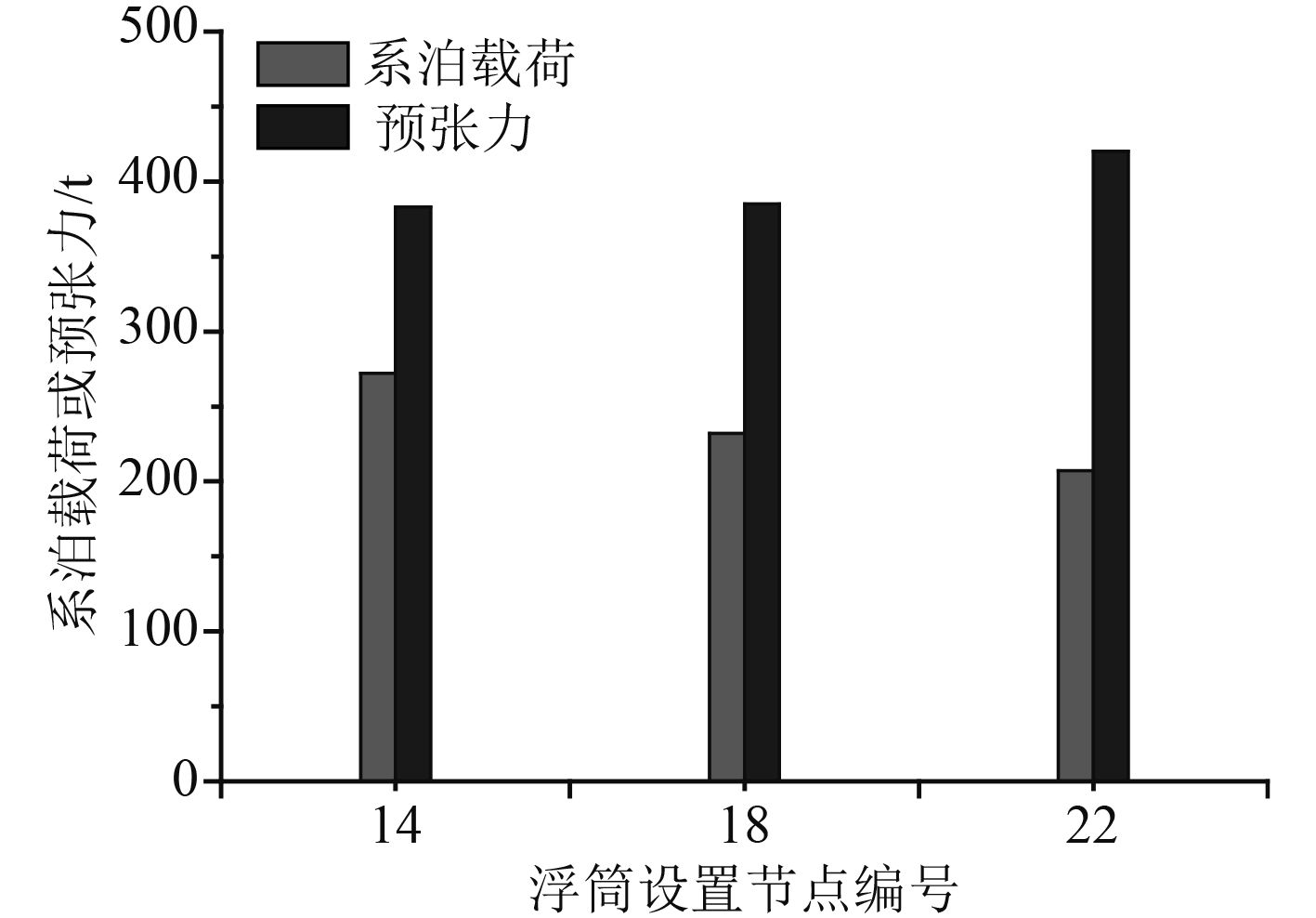图 10 预张力与系泊载荷的变化曲线 Fig. 10 Curves of mooring load and pretension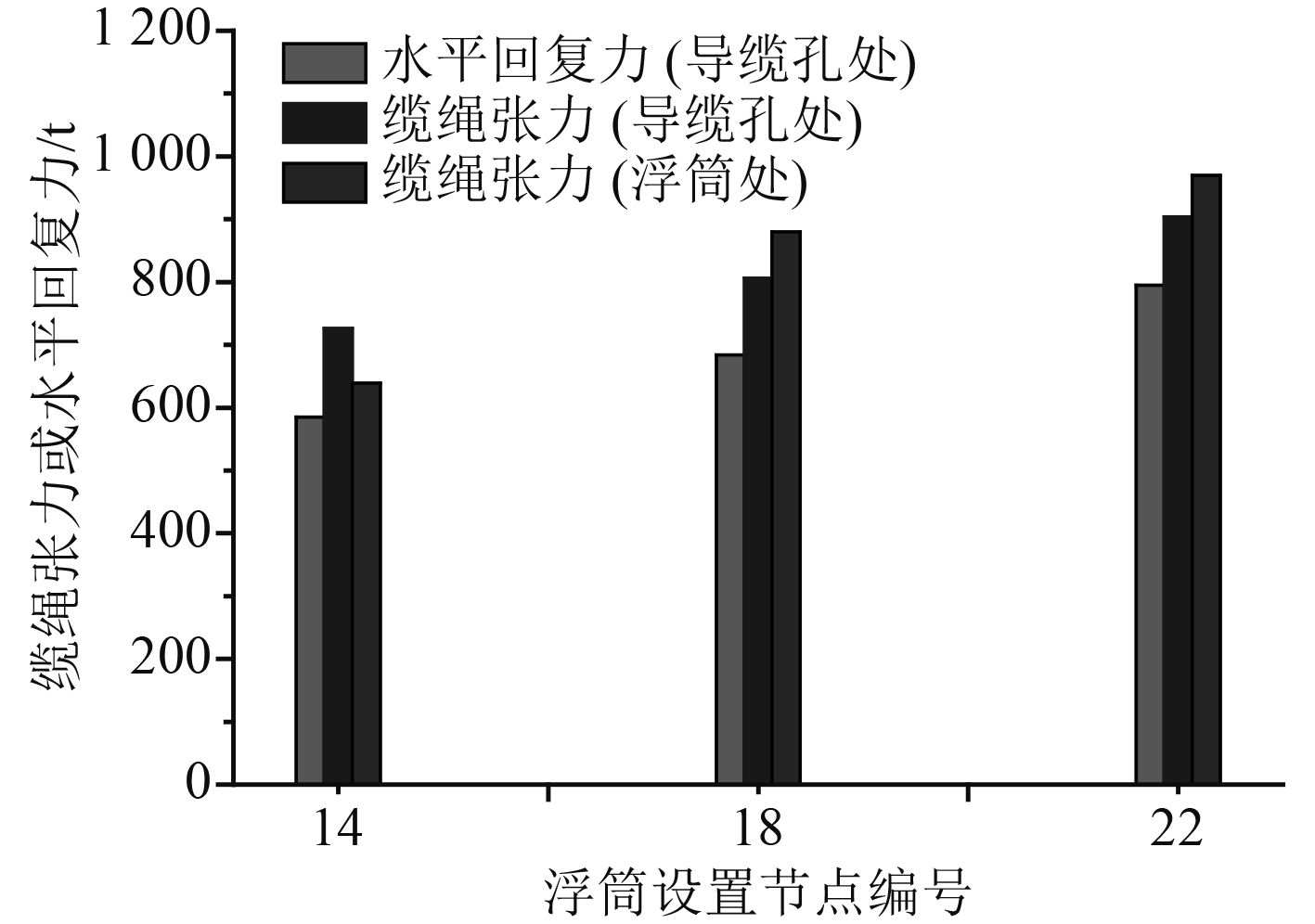图 11 缆绳张力的变化曲线 Fig. 11 Curves of tension at different location

2.3 浮筒海流力的影响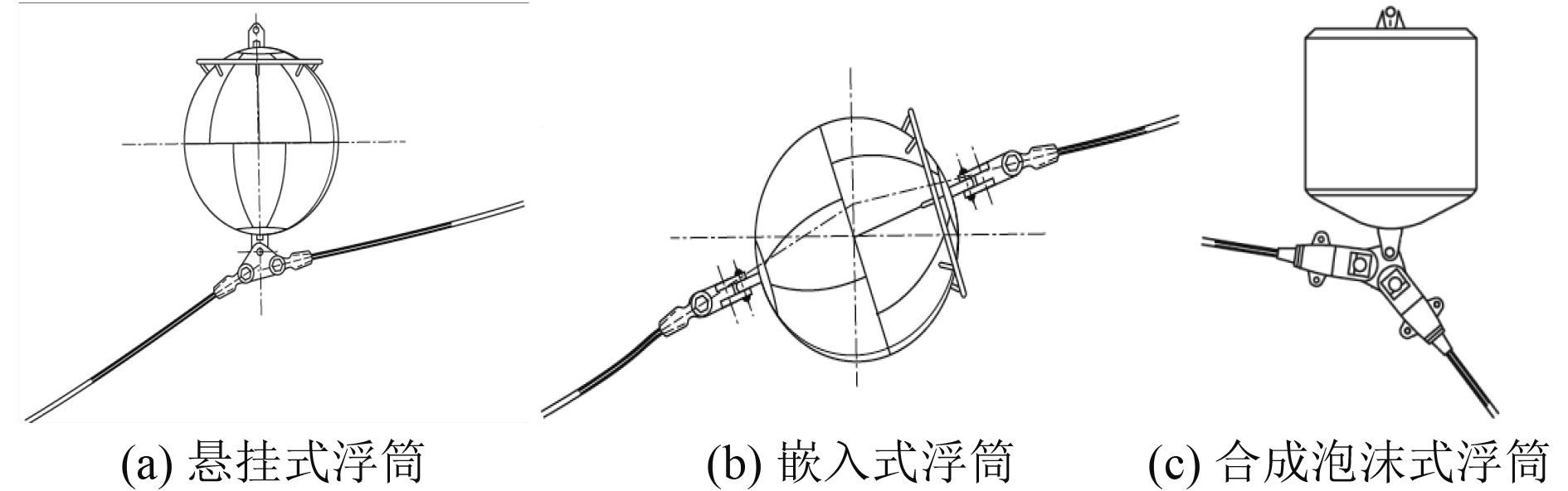图 12 常见的水下浮筒形式 Fig. 12 Normal submersible buoy configurations

 ${F_{cs}} = {\text{515}}{\text{.62 }}\left( {{C_d}{A_c}} \right)V_c^2\;\text{，} \quad {\rm N}\text{。}$ (8)表 3 浮筒水平向海流力计算结果 Tab.3 Result of horizontal current force act on the buoy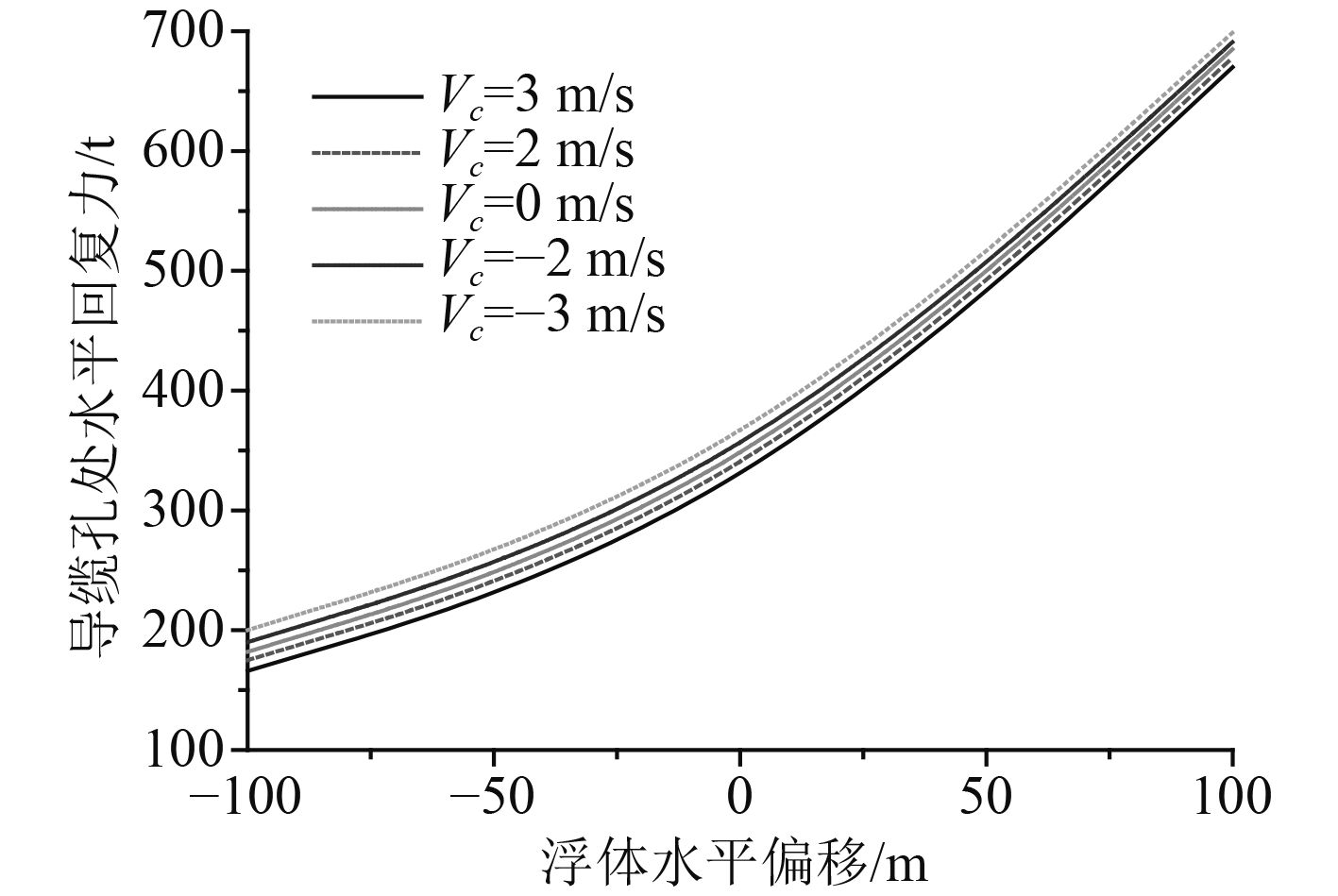图 13 缆绳水平刚度变化曲线 Fig. 13 Curve of horizontal restoring stiffness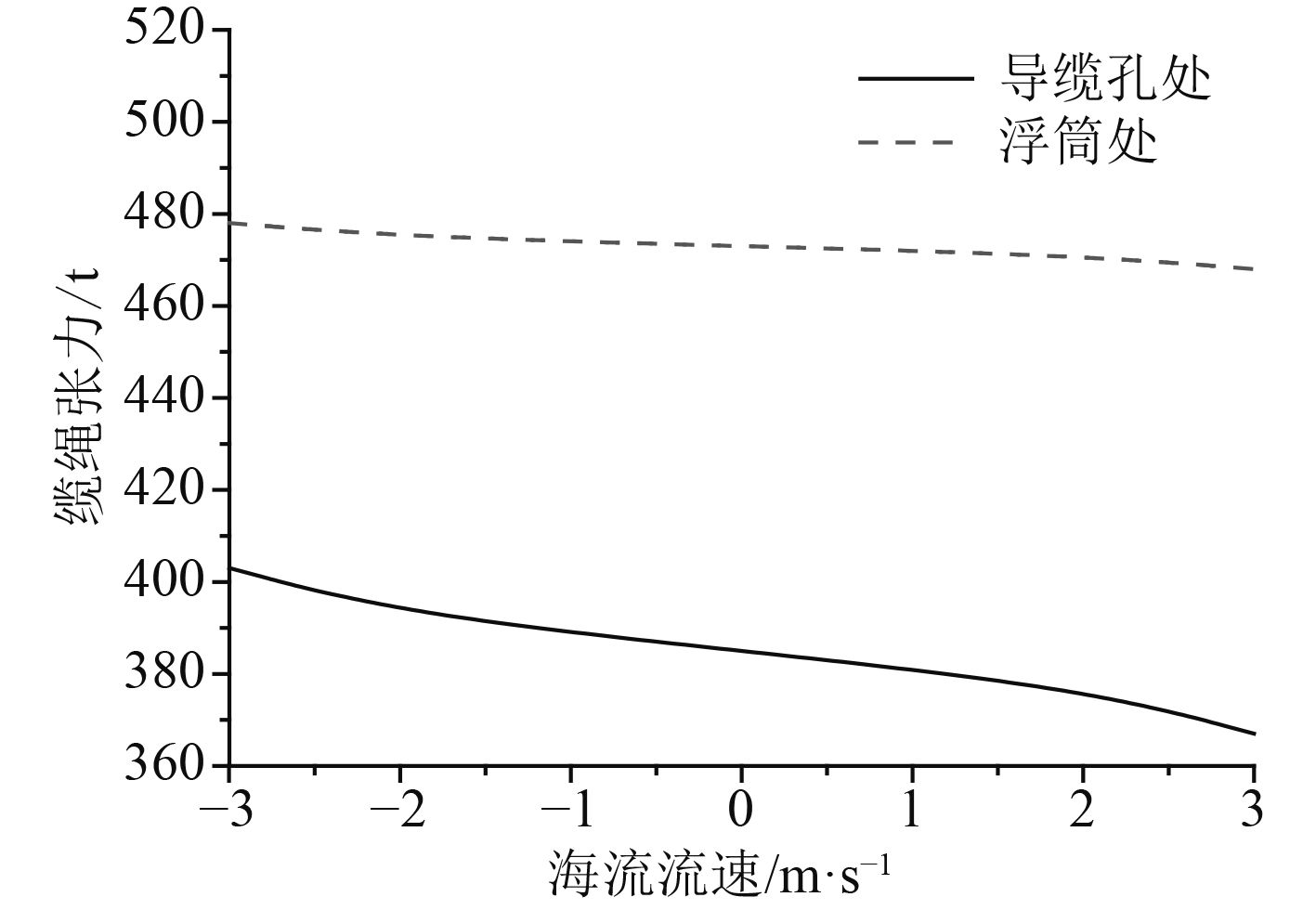图 14 不同位置缆绳张力的变化 Fig. 14 Curve of tensions at different location

3 结　语

1）设置水下浮筒可有效降低系泊载荷与预张力，提高缆绳水平回复刚度，从而起到改善系泊性能的目的，但可能导致最大张力位置的改变，即当平台出现较大水平偏移后，浮筒下端处缆绳的系泊张力上涨很快，有可能超过导缆孔成为整根缆绳强度校核的制约因素。

2）水平恢复刚度方面，在给定缆绳规格与浮筒位置的前提下，浮筒的净浮力将存在最优值，此时缆绳所需施加的预张力最低，但水平恢复性能却最强，此外缆绳不同位置处的系泊张力也最为均衡；浮筒位置也会对缆绳的静力特性产生明显影响，若想提高系泊刚度，应使浮筒位置靠近导缆孔处，若想控制系泊张力，则应使浮筒尽量位于缆绳的中部位置。

3）常规海流流速下浮筒受到的水平向海流力远小于净浮力，因此若采用悬挂式水下浮筒，则水中浮筒基本可保持正浮状态，海流流速对于缆绳静力特性的影响程度也较小。

  李志海, 徐兴平, 黄慧丽. 海洋平台系泊系统发展[J]. 石油矿场机械, 2010, 39(5): 75–78.  由纪昆. 新型深水系泊技术[J]. 中国水运, 2008, 8(2): 34–35. http://doi.wanfangdata.com.cn/10.3969/j.issn.1006-7973-C.2008.02.016  王言英, 肖越. 深水锚泊的新概念与新技术[J]. 船舶工程, 2004, 26(2): 1–3.  HASLUM H A, TUE J. Red hawk polyester mooring system design and verification[C]//Offshore Technology Conference Proceedings. Houston: Offshore Technology Conference, 2005.  American petroleum institute. Design and analysis of station keeping systems for floating structures[S]. API Recommended Practiced 2SK, 2005.  陆中杰, 周国平. 深水锚系泊作业技术应用初探[J]. 船舶设计通讯, 2011(Z1): 67–72. http://www.wanfangdata.com.cn/details/detail.do?_type=perio&id=cbsjtx2011z1013  黄勇. 系泊浮筒的设计与动力分析[J]. 中国水运, 2009, 9(1): 5–7.